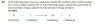• ElegantSir

## Homework Statement

I have the question with a diagram posted in the thumbnail to make things easier.

E = mc²

## The Attempt at a Solution

The homework only had a single example for a question like this so I am not 100% sure how to get the answer. So far I've added the 2 masses:
5.030151g and 5.011267g

Then I subtracted them both 5.030151 – 5.011267 = 0.018884g

Here is where I am stuck. I know that from the example I need to do something like 1.8884 * 10^(input some number here) but I have no clue how to get the number that it should be to the power to. All I know is after this I need to multiply 1.8884 * 10 ^ (?) by 3.8 * 10 ^ 8 to calculate the total amount of energy produced using E = mc²

#### Attachments

•Untitled.png
7.2 KB · Views: 358

## Homework Statement

I have the question with a diagram posted in the thumbnail to make things easier.

E = mc²

## The Attempt at a Solution

The homework only had a single example for a question like this so I am not 100% sure how to get the answer. So far I've added the 2 masses:
5.030151g and 5.011267g

Then I subtracted them both 5.030151 – 5.011267 = 0.018884g

Here is where I am stuck. I know that from the example I need to do something like 1.8884 * 10^(input some number here) but I have no clue how to get the number that it should be to the power to. All I know is after this I need to multiply 1.8884 * 10 ^ (?) by 3.8 * 10 ^ 8 to calculate the total amount of energy produced using E = mc²
Why are you assuming that the masses of the elements reacting are not in grams?

I think you are assuming, based on the wording of the question, that only 1 atom of D and T react, but that need not be the case. Only the ratios of the reactants matter.

Also, units should be carefully scrutinized here. If you take c in m/s, what mass units must you use in order to calculate energy in joules?

Why are you assuming that the masses of the elements reacting are not in grams?

I think you are assuming, based on the wording of the question, that only 1 atom of D and T react, but that need not be the case. Only the ratios of the reactants matter.

Also, units should be carefully scrutinized here. If you take c in m/s, what mass units must you use in order to calculate energy in joules?
Im not quite sure what you mean by all of that. I assume you mean I need to convert grams to a different unit of measurement but I am not sure what. Also what do you mean by
"I think you are assuming, based on the wording of the question, that only 1 atom of D and T react?"

Im not quite sure what you mean by all of that. I assume you mean I need to convert grams to a different unit of measurement but I am not sure what.

Well, joules are the SI units of energy. Joules are also derived units. What is the definition of a joule?

https://en.wikipedia.org/wiki/Joule

Also what do you mean by
"I think you are assuming, based on the wording of the question, that only 1 atom of D and T react?"

It's obvious that 1 atom of deuterium does not have a mass of 2.014102 g, but a certain number of deuterium atoms does have this total mass. Ever heard of a fellow by the name of Avogadro?

If you're going to study physics, you've got to study units and understand how units are useful in deciphering physics problems.

Well, joules are the SI units of energy. Joules are also derived units. What is the definition of a joule?

https://en.wikipedia.org/wiki/Joule

It's obvious that 1 atom of deuterium does not have a mass of 2.014102 g, but a certain number of deuterium atoms does have this total mass. Ever heard of a fellow by the name of Avogadro?

If you're going to study physics, you've got to study units and understand how units are useful in deciphering physics problems.
Ahh I see where I went wrong. The example

Ahh I see where I went wrong. The example questions like to use numbers like 1.9 * 10^-4 rather than use the actual whole number so I got confused.

I would need to convert grams to kilograms which would mean the number would now become: 1.8884 * 10^-5
Now I plug it into E = mc^2 and get:

E = 1.8884 * 10^-5 * (3 * 10^8)^2
E = 1.7 * 10 ^ 12 (rounded of course)

Im assuming this is the correct answer now?

Ahh I see where I went wrong. The example questions like to use numbers like 1.9 * 10^-4 rather than use the actual whole number so I got confused.

I would need to convert grams to kilograms which would mean the number would now become: 1.8884 * 10^-5
Now I plug it into E = mc^2 and get:

E = 1.8884 * 10^-5 * (3 * 10^8)^2
E = 1.7 * 10 ^ 12 (rounded of course)

Im assuming this is the correct answer now?
Always include the units in your calculation results. That's a habit you should acquire now.

Always include the units in your calculation results. That's a habit you should acquire now.
Ahh yes it would be in Joules...Thanks for the help!